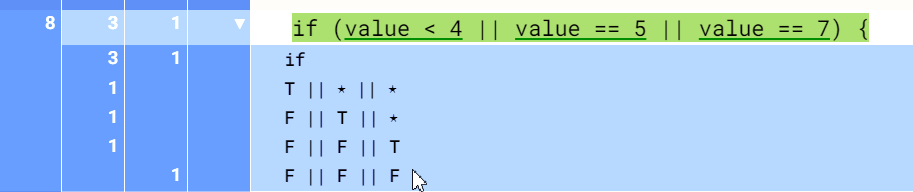How Can We Help?

# Why You Need Code Coverage Metrics

Code coverage metrics are used to measure the degree to which the source code of a program has been tested.

We need to measure the effectiveness of our tests. The best way we do this is by looking at coverage metrics. Coverage metrics can tell us how thoroughly we’ve tested a particular area of code and identify areas that still need testing.

## Code Coverage Types

### Function Coverage:

Function coverage is an important part of quality assurance and quality control, as it ensures that all functions within an application or system have been defined and tested.

Function coverage gives you a quick overview of the number of times functions are executed. RKTracer code coverage tool tracks and show the number of times the function/method was executed in testing.

Here isprime function was executed twice in dynamic testing.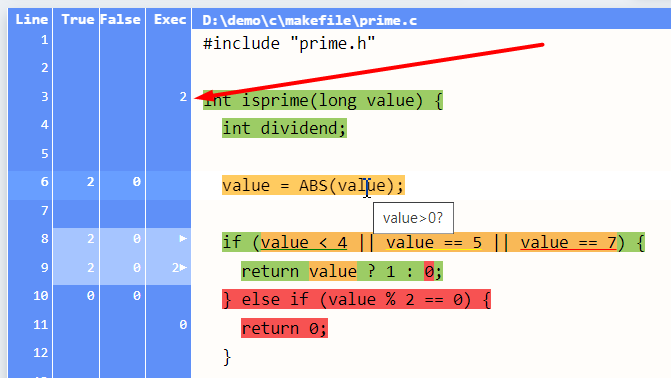### Line Coverage

Line code coverage is a measure of how much of the code in a program has been tested. It is computed by dividing the number of lines executed (or touched) by the number of lines in the program.

Line Coverage= Number of lines of code executed during testing/Total number of executable lines of code

In most programming languages, line coverage is not equal to statement coverage.

for loop has three statements:

for (dividend = 3; dividend <= value/dividend; dividend+=2)

Statement 1 : for (dividend = 3; dividend <= value/dividend; dividend+=2)

Statement 2 : dividend = 3;

Statement 3 : dividend <= value/dividend;

Line coverage is essential because it provides you with a bird’s-eye view of the total lines of code covered in testing. In a single line, there are usually two or more statements. The RKTracer tool will highlight which parts of code or conditions have been covered.

• Green color = Covered
• Yellow color = partially covered
• Red color = Not covered

The RKTracer tool lets you know the summary of line coverage at different levels for better analysis i.e., function, file, and project. We can see all three colors in a single source code line based on conditional expressions executed. Line coverage is dependent on the assessment of other formats of the code and hence is less reliable.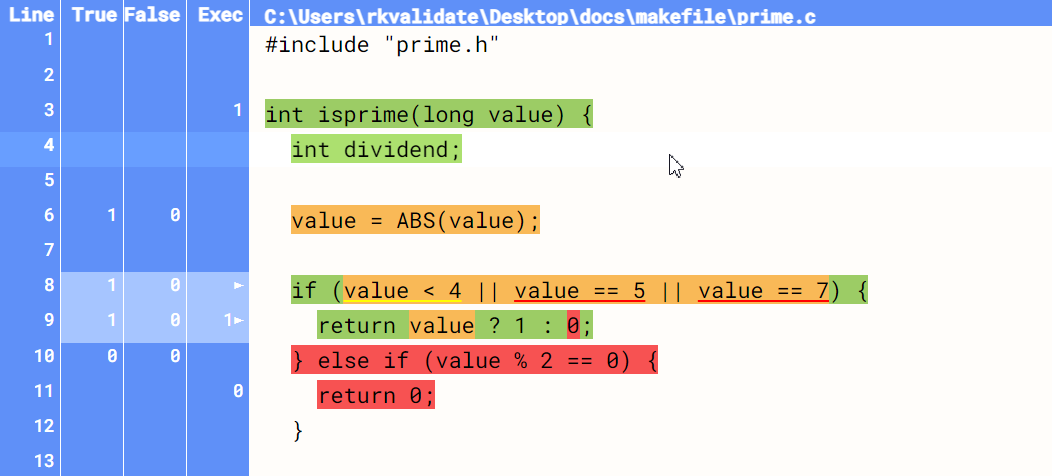### Statement Coverage

Statement code coverage is one of the most popular metrics for measuring code coverage. It refers to the percentage of statements of code that are executed by the tester on average per statement executed.

For EX: variables, loops, conditions like “if,” “while,” “for,” or simple statements like a semicolon which is executable code, or anything ending with a semicolon is considered as a statement. Any empty statement with no machine code just ‘”;” a semicolon is not considered a statement.

Statement Coverage= Number of statements executed during testing/Total number of executable statements in an application

Statement coverage is not equal to line coverage in most programming languages. However, vice versa is impossible as the line contains multiple statements.

In programming languages like C, C++, Java, and C#. In a single line, we can have more than one statement or condition, or branch. In these cases, some might be executed, some might be partially executed, and some may not have been executed at all in testing

a++; b+=2; if (a) b=0;    //Here we have four statements in a single line.

• statement 1 a++;
• statement b+=2;
• if-statement: if (a) b=0;
• statement b=0;

### Branches Coverage

Decision coverage is also referred to as branch coverage. This means that each condition should be executed at least once.

If (a>b || b>c )

{

else

}

If the if and else statements have been executed once to true and once to false consecutively, we will have 100% branch coverage. Here sub-conditions (a>b and b>c) are ignored. However, the Boolean expressions are ignored.

### Condition Coverage

Condition coverage is also termed as expression coverage metric. Every sub-expression must be at least once to true and once to false.

If (a>b || b>c )

{

else

}

Condition coverage is similar to branch coverage but also checks for individual sub-expression(a>b and b>c) outcomes for turn and false. It is used to check individual outcomes for each logical condition. However, the complete coverage in condition coverage does not imply a total branch/decision coverage rate.

### MC/DC Code Coverage

Every sub-condition should be executed at least once to true and once to false in a given decision block. As a result, the outcome of the decision block should be changed to either true or false.

Decsion block “if (value < 4 || value == 5 || value == 7)”  complete if condition is decision block

sub-condition 1: value < 4

sub-condition 2: value ==5

sub-condition 3: value ==7

Let’s say we have following decision “if (value < 4 || value == 5 || value == 7)” block with three sub-conditions i.e “value < 4”, “value == 5” , “value == 7”. If we pass value==3 then sub condition “value<4” will be executed for true ,as value 3 is less then 4.However MC/DC states sub condition should be executed atleast once to true and once to false and as a result of it decision block should be changed to either true or false. Since “value<4” has been executed only to true ,so MC/DC condition has not met.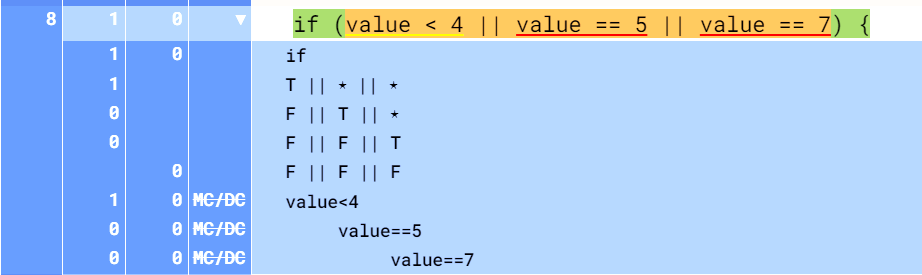Let’s say we pass two values, i.e., value==3 and then value==4, so that the sub-condition “value<4” will be executed for once to true and one to false as a result decision block will be executed to true or false condition.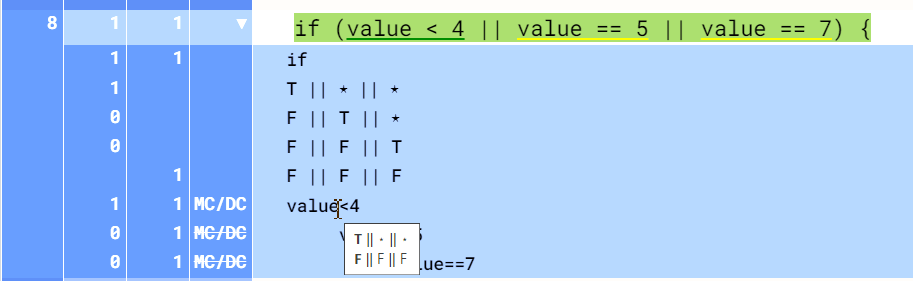You can see that we have covered MC/DC coverage for sub condition “value<4,”  and you need to take the same approach for the remaining two MC/DC conditions.

### Multiple Condition Coverage

It’s also known as a combination of condition coverage. Multiple condition coverage performs tests for every true/false value with the combination. Multiple condition coverage is done for every individual condition in the decision. The coverage is sought by dividing the total number of successful condition combos and line blocks by the total number of conditions and blocks in the project.

Multiple condition coverage is the most efficient and reliable coverage metric when the coverage rate is entirely in multi-condition coverage. You can be assured that the coverage is complete in all other covers.

There are four multiple condition coverage points covered in a given decision block if we pass value==3 to a given “if” block. Only one condition is covered.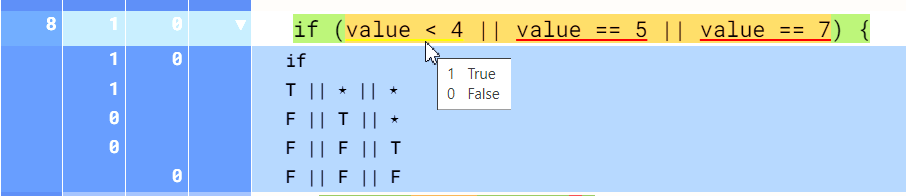Now let’s pass values 3,5,7, and 89. Now we can see that all the multiple conditions points are covered.